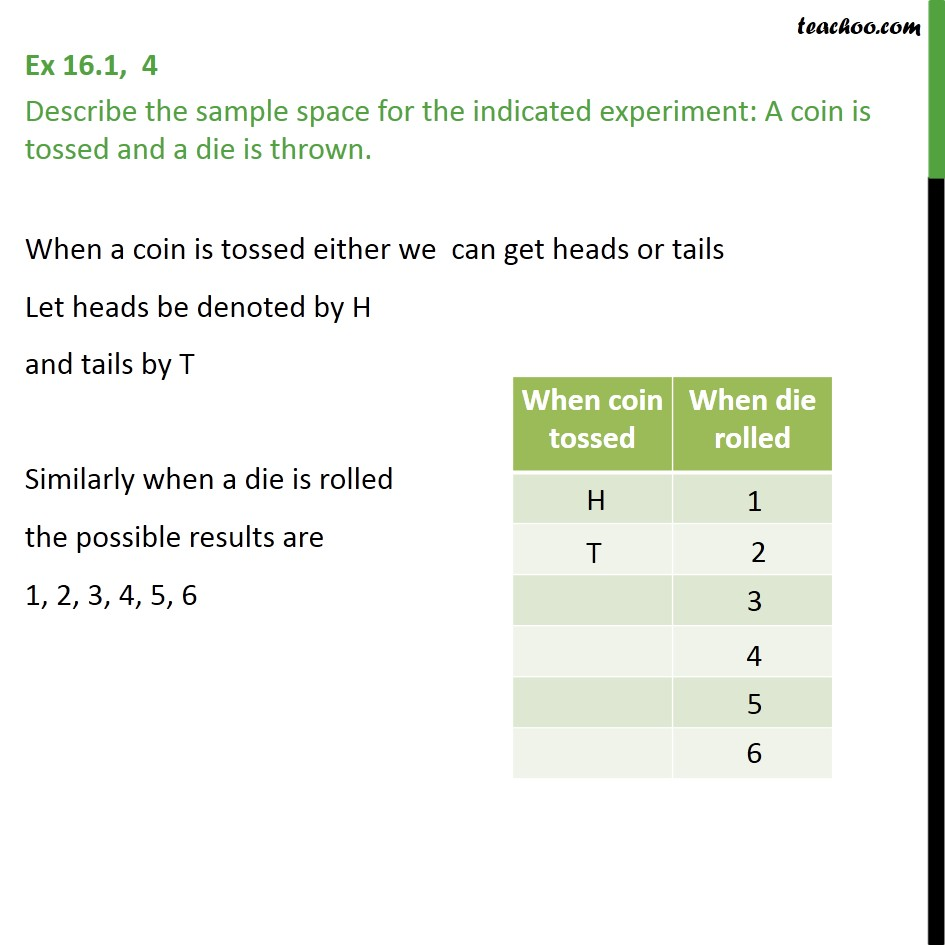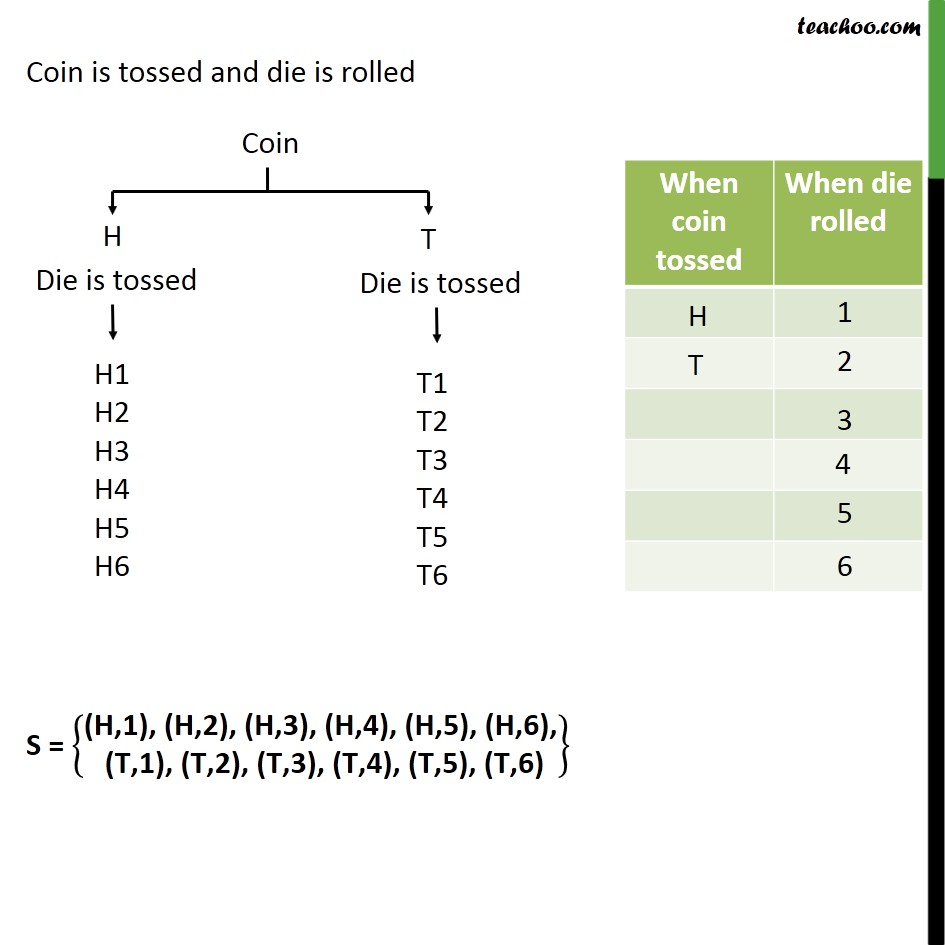Subscribe to our Youtube Channel - https://you.tube/teachoo

1. Chapter 16 Class 11 Probability
2. Concept wise
3. Sample Space

Transcript

Ex 16.1, 4 Describe the sample space for the indicated experiment: A coin is tossed and a die is thrown. When a coin is tossed either we can get heads or tails Let heads be denoted by H and tails by T Similarly when a die is rolled the possible results are 1, 2, 3, 4, 5, 6 Coin is tossed and die is rolled S = (H,1), (H,2), (H,3), (H,4), (H,5), (H,6), (T,1), (T,2), (T,3), (T,4), (T,5), (T,6)

Sample Space